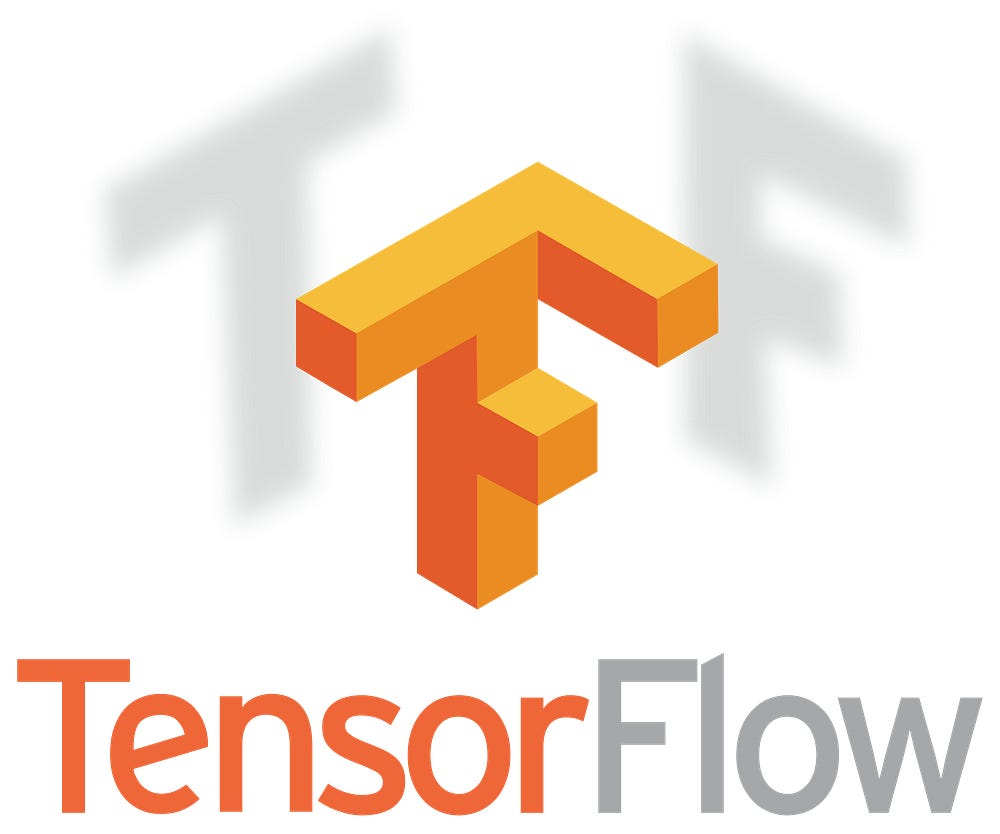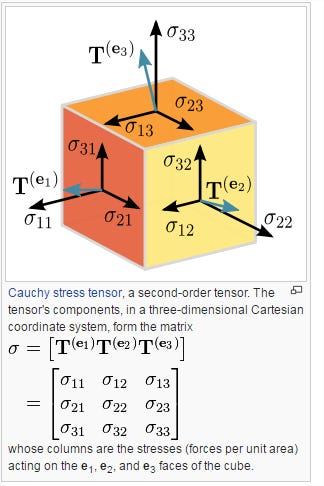# Series: Learning Tensorflow the easy way

This is part 1 of a series of articles on how to get started with TensorFlow.

Given the recent spike in interest in the deep learning field, there is often a fiery debate that happens among data scientists — which framework is the best? This, like so many other things in this field, is entirely subjective.

But one framework, that most experts like and use, is Google’s TensorFlow™. It’s the most widely used framework by industry experts and researchers, it represents a good starting touch point for newcomers to deep learning.Tensorflow logo

TensorFlow™ is a flexible architecture that allows easy deployment of machine learning computation across a variety of platforms (CPUs, GPUs, TPUs), from desktops to clusters of servers to mobile and IoT devices.

In this series, we shall cover the bread and butter of Tensorflow framework with lots of interesting visualizations and side-projects to work on.

### Prerequisites

To make the most out of this tutorial, we expect the following prerequisites are met:

1. Basic understanding of Python
2. Basics of Machine Learning
3. Familiarity with Jupyter Notebooks

We will cover the progress of Learning Tensorflow the easy way in the following parts;

Part 1: Tensorflow Installation and Setup, Syntax, and Graphs

Part 2: Variables and Placeholders in Tensorflow and Operations

Part 3: Implementing a Regression Example in Tensorflow

Part 4: Implementing a Classification in Tensorflow

### Installation and Setup:

Tensorflow supports the following 64-bit opertating systems.

• Ubuntu 16.04 or later
• Windows 7 or later
• macOS 10.12.6 (Sierra) or later (no GPU support)
• Raspbian 9.0 or later

The pip installation requires Python 2.7, 3.4, 3.5, or 3.6. You may check your Python and pip version by using the following commands:

``python3 --version``pip3 --version``

It should output the your python and pip versions. It is recommended that you setup a virtual environment. I have anaconda installed that supports many dependencies of tensorflow. We can proceed with a conda command to create a python 3.5 virtual environment.

``conda create -n py35 python=3.5``

This should take a while. Now, you can switch to environment using `source activate py35`

Once, you are in your newly created environment, you can install tensorflow with pip command;

`pip install -- tensorflow==1.3`

Let’s open up a jupyter notebook using `jupyter notebook` command and import tenforflow using the following command.

`import tensorflow as tfprint("Tensorflow version: {}".format(tf.__version__))`
`Output: Tensorflow version: 1.3.0`

Note that this tutorial is made on 1.3.0 version and the syntax might differ if you use any previous version of tensorflow.

### Tensorflow Basics: Syntax and Graphs

#### Understanding the “Tensor” in TensorFlow

Tensors are simply arrays of numbers, or functions, that transform according to certain rules under a change of coordinates. We typically see them in relations such as the dot product, the cross product, and linear maps.

Now, as previously mentioned that tensors are arrays of numbers. But, it should be noted that Tensors are not another name for multi-dimensional arrays. There is a significant difference between Tensor and multi-dimensional arrays. Simply put, multi-dimensional arrays are data structures for representing tensor in a coordinate system.Cartesian Tensor

#### The story of Graphs in Tensorflow

The Tensorflow works on the tensors and their flow of operations through a computation graph. Hence the name; Tensor+flow = Tensorflow!

A computational graph is a series of TensorFlow operations arranged into a graph of nodes.

At first we create a model which is a computation graph with tensors as objects. Then we create a session in which we run the computation all at once. Don’t worry if the concept is glitchy here. Let us implement the same in code to understand it better

`import tensorflow as tf`
`first_string = tf.constant('Analytics')second_string = tf.constant(' Vidhya')`

Now, if you check the type of either variables with `type(first_string)` command. You should see that it is a Tensor object not a string.

`Out []: tensorflow.python.framework.ops.Tensor`

Now, lets’ try and add both the strings and try to print it. The type remains the same. But, the output is not what is usually expected.

`combined_string = (first_string + second_string)print (type(combined_string))print (combined_string)`
`Out []: <class 'tensorflow.python.framework.ops.Tensor'>        Tensor("add:0", shape=(), dtype=string)`

This is how computational graph works. The `combined_string` is not directly the result of addition of two strings but is just an operation to be carried out. On this very basis the tensorflow works. First you define a path and later on execute your pipeline in a session.

Now, to see the expected results we have to run the session for the computation to happen. You can run it using the following command;

`with tf.Session() as sess:    result = sess.run(combined_string)    print (result)`
`Out []: b'Analytics Vidhya'`

We use `with tf.Session()…` as it automatically opens and closes the session after running the computation for us.

To get a hang of it, lets try out some matrix operations in a bulk code that take two matrices as input and then multiply them.

`Matrix_1 = tf.constant([ [1,3],                          [2,6]])Matrix_2 = tf.constant([ [13,26],                          [23,52]])print("The Shape of Matrix 1 is {} and of Matrix 2 is {}".format(Matrix_1.get_shape(), Matrix_2.get_shape()))`
`MatMul = tf.matmul(Matrix_1, Matrix_2)`
`with tf.Session() as sess:    result = sess.run(MatMul)print(">> Output")print(result)`
`Out []: The Shape of Matrix 1 is (2, 2) and of Matrix 2 is (2, 2)        >> Output        [[ 82 182]         [164 364]]`

So in general you need to remember the following things:

1. Graphs are set of nodes
2. The connections are referred as edges
3. In Tensorflow, each node is an operation with some inputs that supplies output after execution

That’s all for this introductory part. The Jupyter notebook is available on GitHub here. We shall be covering the next concepts in

Part 2: Variables and Placeholders in TensorFlow and Operations

Stay tuned. And, don’t forget to initialize your tensors. For any help or suggestion, do use the comment section below.

Source: Deep Learning on Medium